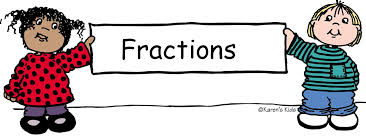# Maths

:This term the children have been re-visiting place value. We have reminded ourselves what each digit represents.What is place value? - BBC BitesizeQuick Quizz
What is the value of the 4 in the number 549,632?

1.hundred thousands
2.ten thousands
3.40,000
4.400,000

Comparing whole numbers to 100000 with three decimal placesLet's look at how to compare and order decimals, using number lines to help us.

## Example 1

On the number line below we can see that 2.3 (two and three tenths) is less than 2.8 (two and eight tenths).

2.8 is five tenths more than 2.3.

Comparing and ordering decimals - Maths - Home Learning with BBC Bitesize - BBC BitesizeRounding to nearest 10,100,1000,100000Finding powers of 10,100,1000 moreOnline games your children might enjoy...Hit the Button - Quick fire maths practise for 6-11 year olds (topmarks.co.uk)

What is column addition? - BBC Bitesize

This term we have been learning about multiples and factors...

What are multiples? - BBC Bitesize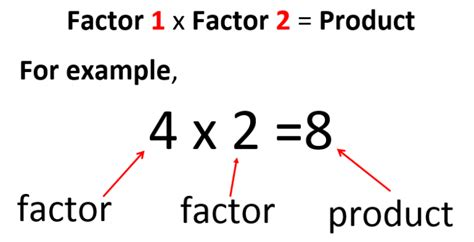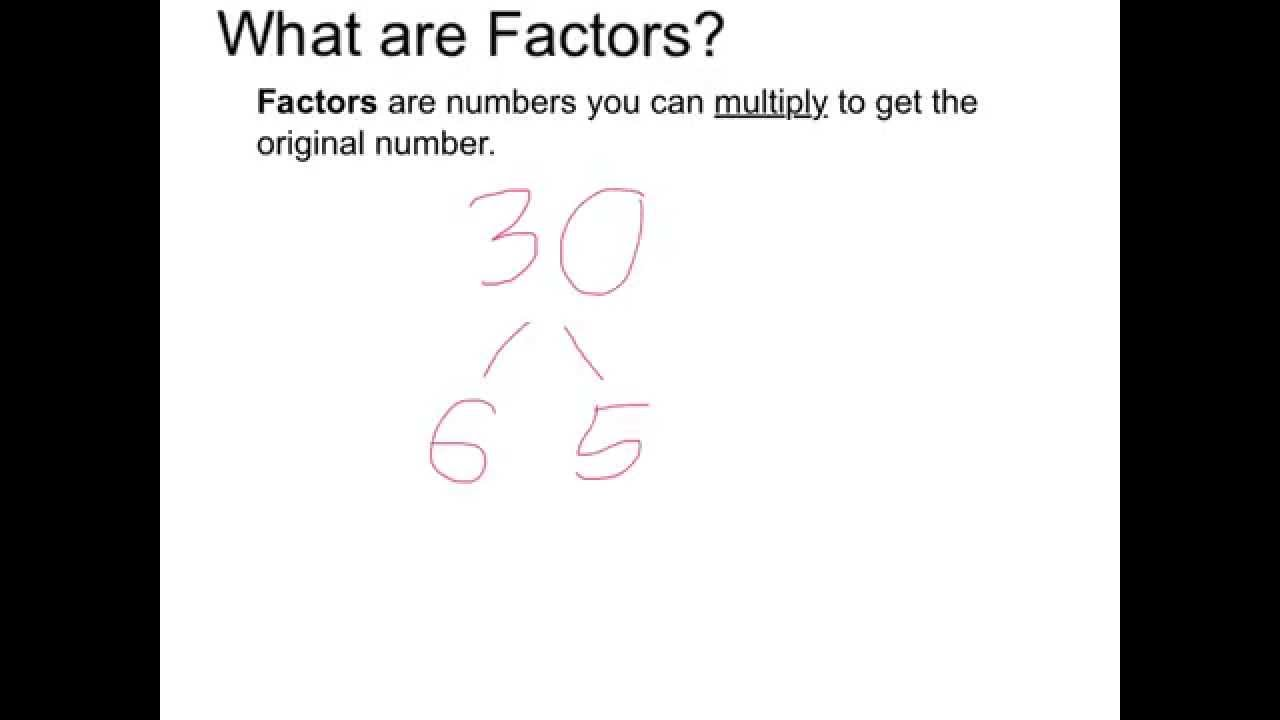Click the link below to play TTRockstars.

https://ttrockstars.com/

Today we are looking at common factors.

• common factor is a number that can be divided into two different numbers, without leaving a remainder.
• Often numbers can share more than one common factor.
• It is possible to find the common factors of more than two numbers. Please click the link below and watch for further understanding.

https://www.bbc.co.uk/bitesize/topics/z6j2tfr/articles/z72r97hToday the children are learning all about Square Numbers.The children really enjoyed today's lesson.

Please click on the BBC Bitesize logo for further understanding.This week we have also been practicing our bus stop method for division.Create a fact box to help you. Then you can start subtracting by using your fact box.

Click on the link below for further understanding.

The children have been really busy learning the chunking method. They are building their confidence daily by putting the techniques they have learned in word problems.This week the children will be learning Fractions.

What are Fractions?Ordering Fractions and equivalent Fractions this week.This week the children are studying multiplication, 3 digits multiply 1 digit.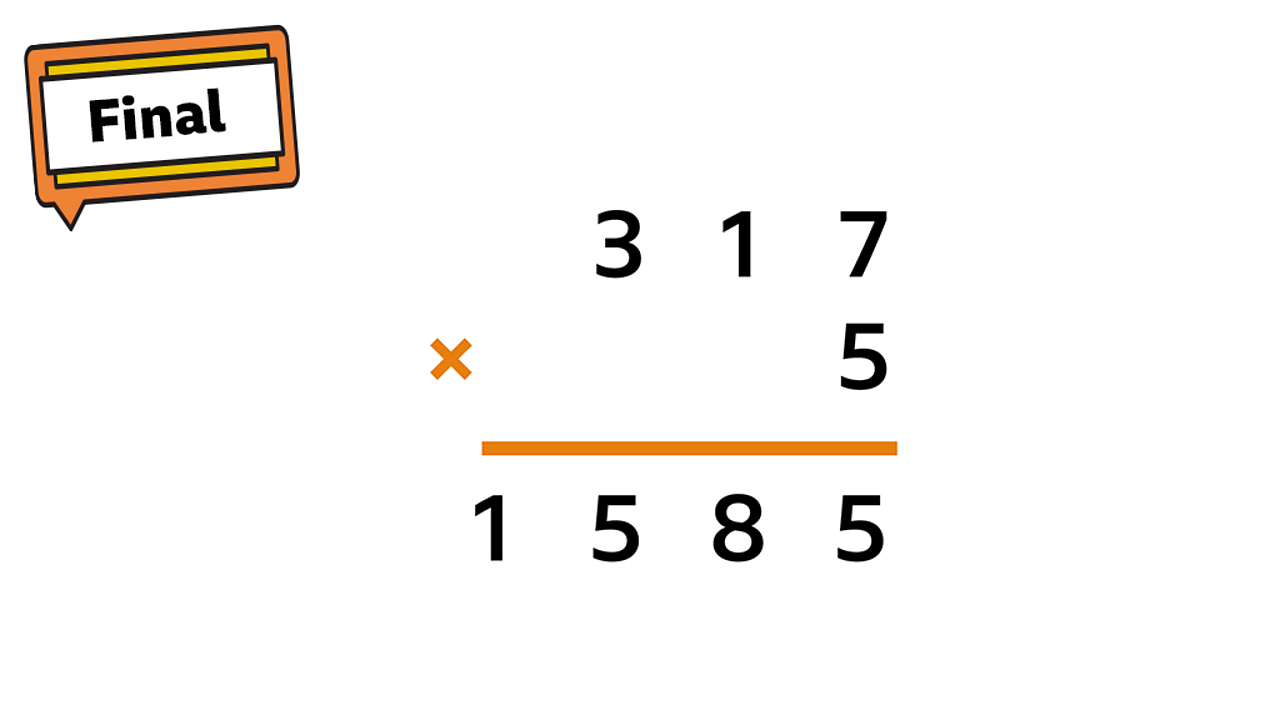Multiply a 3-digit number by a 1-digit number - Maths - Learning with BBC Bitesize - BBC Bitesize

Spring 1This term the children are learning to tell the time, digitally and anologue.

https://www.bbc.co.uk/bitesize/topics/zkfycdm/articles/zcrmqty

Reflection

When light from an object is reflected by a surface, it changes direction. It bounces off the surface at the same angle as it hits it.

Smooth, shiny surfaces such as mirrors and polished metals reflect light well. Dull and dark surfaces such as dark fabrics do not reflect light well.What is reflection? - BBC Bitesize

# Reflection

 Reflections are everywhere ... in mirrors, glass, and here in a lake. ... what do you notice ?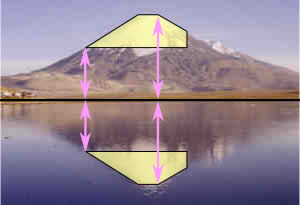Every point is the same distance from the central line !
... and ...
The reflection has the same size as the original image
The central line is called the Mirror Line ...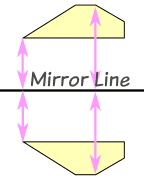## Can A Mirror Line Be Vertical?

Yes.
Here my dog "Flame" shows a
Vertical Mirror Line (with a bit of photo magic)
 In fact Mirror Lines can be in any direction.Imagine turning the photo at the top in different directions ...... the reflected image is always the same size, it just faces the other way: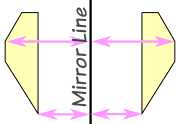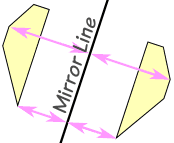WEIGHT in Grams & KilogramsThese are the most common measurements:
• Grams
• Kilograms
• Tonnes
Grams are the smallest, Tonnes are the biggest.
Let’s take a few minutes and explore how heavy each of these are.
 1 kilogram = 1,000 grams A dictionary has a mass of about one kilogram.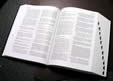Kilograms are great for measuring things that can be lifted by people (sometimes very strong people are needed of course!).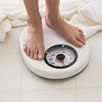Kilograms are often written as kg (that is a "k" for "kilo" and a "g" for "gram), so "10 kg" means "10 kilograms". Scales measure our weight using kilograms. An adult weighs about 70 kg. How much do you weigh?

But when it comes to things that are very heavy, we need to use the tonne. (1000kg = 1 tonne)

First we need to measure or wegh an object & then we can calculate either grams or kilograms.Multiplying and dividing decimals

Decimals are used every day, for example, when using money. Knowing how to use decimal points and places when adding, subtracting, dividing and multiplying is an important mathematical skill.

What are fractions? Do we need to learn about them? HMMMM... Lets take a look!